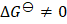# Select the correct statement a) There is a natural asymmetry between converting work to heat and converting heat to work b) No process is possible in which the sole result is the absorption of heat from a reservoir and its complete conversion into work c) For every chemical reaction at equilibrium, standard change in Gibbs free energy is zero d) At constant temperature and pressure, chemical reactions are spontaneous in the direction of decreasing Gibbs energy

## Question ID - 103433 :- Select the correct statement a) There is a natural asymmetry between converting work to heat and converting heat to work b) No process is possible in which the sole result is the absorption of heat from a reservoir and its complete conversion into work c) For every chemical reaction at equilibrium, standard change in Gibbs free energy is zero d) At constant temperature and pressure, chemical reactions are spontaneous in the direction of decreasing Gibbs energy

3537

 (b,d)ve for spontaneous process andat equilibrium. Statement (b) represents Carnot theorem